# Selecting the Best Data Converter for a Given Noise Budget: Part 3

### Abstract

The practical use of industry data sheets is demonstrated to determine what system specifications can be achieved, in the presence of system errors, such as data-converter quantization, clock jitter, channel nonlinearity, and input- and output-referred noise. Design tools that aid in the analysis of these parameters, and constructive ways to control and reduce other system noise elements are highlighted.

A similar version of this article appears on EDN, December 14, 2013.

#### Introduction

This is the last of a three-part series on noise in the signal chain. In Part 1 about Annoying Semiconductor Noise, we identified the origins and characteristics of semiconductor noise found in all ICs. We explained how it is specified in device data sheets and showed how to estimate the noise of a voltage reference under real-world conditions not specified in the data sheet. In Part 2 on Noise and Distortion in Data Converters, we focused on sources of noise and distortion particular to data converters. We showed how their noise is specified in a data sheet. We conclude in this article by bringing Parts 1 and 2 together. Now we will help readers choose the most appropriate data converter for their noise budget.

#### Noise in the Signal Chain

We begin with a brief review of concepts covered in Part 1 of the series. Noise is any unwelcome electrical phenomenon in an electrical system. Depending on its origin, noise can be classified as external (interference) or internal (inherent) to the signal chain. In Figure 1 all external noise sources are combined into a single term, Vext, and all internal noise sources are merged into a single term, Vint.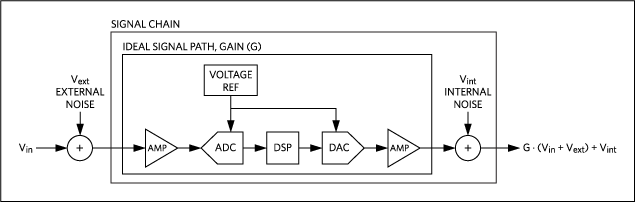Figure 1. Noise in the signal chain.

A noise budget is the allocation of the noise in a signal chain that results in an acceptable signal-to-noise ratio (SNR) at the output. The SNR is defined as the ratio of the full-scale RMS signal level to the total RMS noise. Therefore, to determine the acceptable distribution of noise within a signal chain you must evaluate its effect on total SNR. To this end, two specifications unique to data converters are introduced: signal-to-noise and distortion (SINAD) ratio and effective number of bits (ENOB).

Signal-to-Noise and Distortion
Data converters expand the definition of SNR to include distortion, and use the term signal-to-noise and distortion (SINAD). The added distortion includes all undesired spectral components, excluding DC. SINAD is the ratio of the full-scale RMS signal to the RMS sum of all other noise and distortion components.

SINAD can be expressed in terms of the quantization noise, sample jitter, analog noise, and total harmonic distortion (THD) as: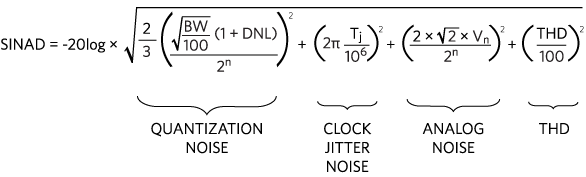(Eq. 1)

Where:
N is the resolution, in bits.
DNL is the average differential nonlinearity, in LSB.
BW is the fraction of the full Nyquist bandwidth used, in percent.
Tj is the ratio of the RMS jitter of the sample period to the period of sine-wave signal, in ppm.
Vn is the analog noise, in LSBRMS.
THD is the total harmonic distortion, in percentage.
SINAD reduces to the familiar, “rule-of-thumb” equation:

 SNR = 6.02N + 1.76dB LSBRMS (Eq. 2)

When:
BW = 100%
DNL = 0 LSB
Tj = 0ppmRMS
Vn = 0LSBRMS
THD = 0%

Together, these parameter values describe the ideal data converter in which the only noise source is the full bandwidth quantization noise inherent in the sampling processes.

In this case, ENOB = N bits.

Effective Number of Bits
Effective number of bits (ENOB) is a measure of an analog-to-digital converter’s (ADC’s) or digital-to-analog converter’s (DAC’s) ability to convert a signal between the analog and digital domains. ENOB is an AC specification and is synonymous with SINAD.

ENOB and SINAD are related by: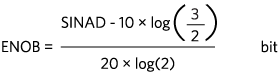(Eq. 3)

ENOB means that a data converter has a level of noise and distortion equivalent to an ideal data converter. That means a data converter with full bandwidth and the equivalent number of bits as the ENOB, but without noise and distortion. ENOB is always less than, or equal to, the resolution (N) of the device. ENOB should not be confused with DC accuracy, which is only a function of resolution (N) and linearity (INL).

#### ENOB Calculator

A free calculator, Effective Number of Bits Calculator (ENOB), is available for making quick work of noise calculations in data converters. To get started, just click on the link and select the Effective Number of Bits (ENOB).

The ENOB Calculator is a program for the HP50g calculator that aids in the design and analysis of ADC and DAC application circuits. Each noise parameter can be entered or found. ENOB can also run on a Windows® PC using the free program HPUserEdit 5.4 found at www.hpcalc.org, or the calculator page.Figure 2. ENOB calculator screen shot.

The ENOB Calculator uses SINAD equations 1 and 3 above, and the noise equations given in Part 2 of this series. Each parameter can be entered or found, so the calculator is useful for both design and analysis. This calculator is used to illustrate a method of selecting the best data converter for a given noise budget. Refer to the User’s Guide (in the zip file with the calculator) for instructions on how to operate the calculator.

#### Selecting the Best Data Converter for Your Noise Budget

A noise budget is the acceptable allocation of noise within a signal chain that yields the desired SINAD. An example best illustrates a step-by-step method for selecting the best data converter for your noise budget. The ENOB Calculator speeds the process because it makes the necessary calculations.

Objective
Select the best ADC given a system requirement of 80dB SINAD when operating with a full-scale signal over a 0kHz to 100kHz bandwidth.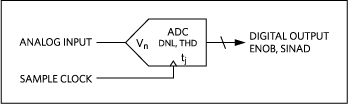Figure 3. ADC with noise-related specifications.

Step 1. Choose the Resolution
Using the simplest Equation 4 for an ideal data converter, we will find the minimum resolution required to meet the SNR: …

 SNR = 6.02N + 1.76dB (Eq. 4)

Solving for N:

 N = (SNR - 1.76)/6.02 (Eq. 5)

Using the ENOB Calculator to make this calculation, we learn that 80db SINAD requires 13 bits of resolution (Figure 4).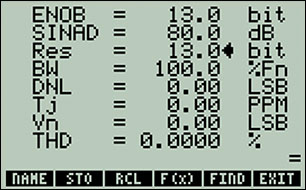Figure 4. The ENOB Calculator determined that 13 bits of resolution are required in our example.

Now select 14 bits. Yes 14 bits and not 13 bits, because real-world ADCs will have a lower SINAD since other factors like DNL, Tj, Vn, and THD are always greater than zero and, thus, increase noise. Entering 14 bits into the calculator, we find that an ADC can provide a SINAD of 86dB (Figure 5).Figure 5. Using 14 bits as your ENOB, the SINAD is 86dB.

This value is greater than the required 80dB, so we start by looking look at 14-bit ADCs.

Step 2. Select an Initial ADC
Find a 14-bit ADC that accepts a 0kHz to 100kHz input signal. A quick search of Maxim Integrated ADCs Parametric Table yields many 14-bit candidates. For this example the MAX1062 is used, and all relevant parameters from the Electrical Characteristics (EC) table in the data sheet are shown in Figure 6.Figure 6. MAX1062 ADC noise parameters.

The parameters relevant to our noise-budget analysis are highlighted in red. The data sheet indicates that this ADC has a typical DNL of 0.5LSB; a typical input-referred noise (Vn) of 0.32LSBRMS; a typical THD of -99dB; and a typical aperture jitter (Tj) of 50ps. In ADCs the input-referred noise is called transition noise because it presents itself as an uncertainty in the transition time between output codes.

Enter the above EC table parameters into the calculator: DNL of 0.5LSB, THD of -99dB, and Vn of 0.32LSBRMS.

In the ENOB calculator Tj is defined as the ratio of the RMS jitter (tj) of the sample clock to the period of a full-scale sine wave, in ppm.

 Tj = (tj/tin) × 106 (Eq. 6)

In this example, the worst-case Tj is found by taking the ratio of 50ps (tj) to the shortest input signal period (tj) of 1/100kHz, and multiplying it by 106. Therefore, Tj = (50 × 10-12/10 × 10-6) × 106ppm = 5ppm.

Enter 5ppm into Tj.

Using the calculator, we learn that SINAD reduces to 80.1dB (Figure 7). The MAX1062 meets our target SINAD of 80dB, with a 0.1dB margin. However, in practice additional margin is needed because we used the typical values from the data sheet instead of the device’s maximum values. We have also not accounted for the presence of any additional noise sources.Figure 7. Now the calculator shows that the SINAD for the MAX1062 is 80.1dB.

Step 4. Examine the Noise Distribution
Before taking steps to reduce noise, we first examine the noise and distortions levels (Figure 8) to see where improvements can be made.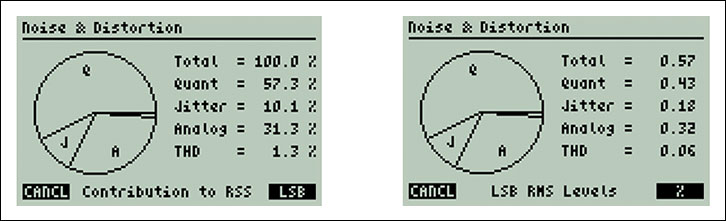Figure 8. The ENOB calculator displays the noise distribution graphically as a percentage of the root sum squared (RSS) or as LSBRMS.

One can see that the quantization noise is the largest contributor to the total noise and distortion. Quantization noise can be reduced by increasing the resolution.

Step 5. Reduce the Quantization Noise
Additional noise margin can be achieved by selecting the 16-bit version of the MAX1162. Again, all relevant parameters appear on the EC table of the data sheet (Figure 9).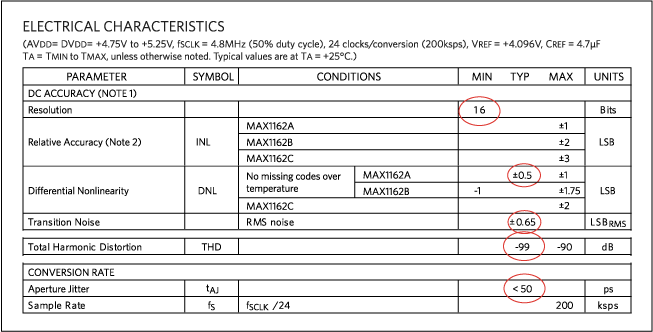Figure 9. MAX1162 ADC noise parameters.

The parameters relevant to our noise budget analysis are highlighted in red. When not given, the typical values are estimated from the 14-bit device, the MAX1062.

Now enter the MAX1162’s parameters into the calculator and find its SINAD:

1. N = 16 bits
2. DNL = 0.5LSB
3. Tj = 5ppm
4. Vn = 0.65LSBRMS
5. THD = -99dB

The MAX1162 SINAD is found to be 86.5dB (Figure 10), which meets our target SINAD of 80dB, with a 6.5dB margin.Figure 10. The MAX1162 is shown to have a SINAD of 86.5dB.

Recall again that typical values were used to predict the SINAD of the MAX1162 In practice, the real value of SINAD may be smaller. You can determine a more conservative estimate by using as many maximum parameter values from the data sheet as possible.

Now we recalculate the SINAD of the MAX1162, but this time using the maximum values in the EC table. This step also helps us to determine whether the MAX1162 will meet our 80dB SINAD requirement using the worst-case DNL and THD. The data sheet indicates a worst-case DNL of 1LSB (max) and worst-case THD of -90dB (max). Entering these values into the calculator, we see that:

1. N = 16 bits
2. DNL = 1.0 LSB
3. Tj = 5ppm
4. Vn = 0.65LSBRMS
5. THD = -90dB

The SINAD is found to be 84.7dB (Figure 11). We conclude that the MAX1162 meets our target SINAD of 80dB with a 4.7dB margin.Figure 11. Using maximum LSB and THD values, the 16-bit MAX1162 is shown to have a SINAD of 84.7dB.

Step 7. Reexamine the Noise Distribution
Reexamining the worst-case noise and distortions levels, we find a generally equal distribution of noise between quantization, sample jitter, input-referred noise, and THD. Note that no single source of noise stands out as a major contributor (Figure 12).Figure 12. The usefulness of the graphic display is emphasized by comparing Figures 8 and 12. The quantizing noise has been reduced dramatically.

The total noise was reduced by 40% from 0.57LSBRMS at 14 bits to 1.35LSBRMS at 16 bits (equivalent to 0.34LSBRMS at 14 bits). This reduction in noise produces the increase in SINAD.

Step 8. Make Some Noise Distribution Trade-offs
Noise can be redistributed between sources within a signal chain (Figure 13) as long as the total noise budget is not exceeded.Figure 13. ADC noise sources within a signal chain.

Clock jitter (Tj) and analog noise (Vn) can have their sources external to the data converter. Therefore, although these specifications are fixed for a given ADC, it may be possible to improve them by changing the circuits external to the ADC. For example, you might use a lower-noise input amplifier and voltage reference or a lower-jitter sample clock.

#### How to Add Random Noise Sources

All uncorrelated noise sources sum geometrically in root sum square (RSS) fashion:

 entotal = √(e2n1 + e2n2 + e2n3 + ... + e2nn) (Eq. 7)

One term often dominates RSS sums. For example, in Figure 14 output noise comes from both the voltage reference (enref) and the DAC (endac).Figure 14. Example of distributed noise sources.

The total output noise is the RMS sum of enref and endac when the DAC output is set to full scale:

 entotal = √(e2nref + e2ndac) (Eq. 8)

If enref = 300nV/vHz and endac = 100nV/vHz, then entotal = 316nV/vHz.

The DAC only contributes 16nV/vHz to the total noise! There is a lesson here: when fighting uncorrelated noise, focus on reducing the dominant terms.

The ENOB Calculator can plot any variable with respect to another. This features is used now to show the possible trade-offs between clock jitter (Tj) and input noise (Vn) without affecting SINAD. Any position on the curve can be selected by the circular cursor, and the parameter trade-offs displayed (Figure 15).Figure 15. The ENOB Calculator compares the trade-off between clock jitter and input noise, with the cursor indicating the trade-off values.

The above cursor position indicates a trade-off between Vn and Tj that maintains a SINAD of 84.7dB. The cursor indicates that if Vn is increased to 0.9LSB, then the clock jitter must be decreased to 2.67ppm to maintain the same SINAD of 84.7dB.

There is, finally, another helpful tool for calculating the error budget in ADC and DAC applications. See the Sidebar below for more details.

#### Conclusion

The typical and maximum values in ACD and DAC data sheets can be used to determine system performance in the presence of noise such as data converter quantization, clock jitter, channel nonlinearity, and input- and output-referred noise. A step-by-step procedure was demonstrated for choosing the best data converter for a given noise budget. An ENOB Calculator aided in the analysis of these parameters, and guided us to constructive ways to control and reduce other system noise elements.

General References

1. Razavi, Behzad, Principles of Data Conversion System Design. IEEE Press, New York, 1995.
2. Maloberti, Franco, Data Converters, Springer, Netherlands, 2008.
3. Maxim Integrated tutorial 4300, “Calculating the Error Budget in Precision Digital-to-Analog Converter (DAC) Applications”.

#### Sidebar: Another Good Signal-Chain Calculator

There is an application note, “Calculating the Error Budget in Precision Digital-to-Analog Converter (DAC) Applications,” that applies equally to ADCs and DACs. It explains how you can use an associated spreadsheet to fill in the blue numbers; the red numbers are calculated for you. With this tool the components' specifications can be traded off against each other to ensure that system specifications are met at the lowest cost. The application note uses data sheet specifications for real parts to illustrate four diverse designs:

1. A consumer audio device in a low-cost, loose-accuracy application
2. A laboratory instrument with high absolute accuracy and precision
3. A one-time-calibrated product with low drift, digital offset, and gain adjustment
4. A low-voltage, battery-powered, moderate-accuracy portable instrument

Each design and the trade-offs involved are discussed to help us understand how to make design decisions. A table is included to compare the error analysis in parts per million (ppm) relative to other DAC system resolutions.